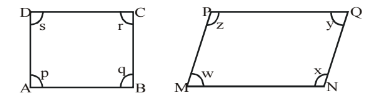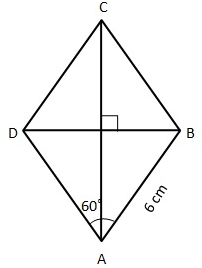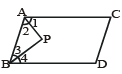Courses

## 25 Questions MCQ Test Olympiad Preparation for Class 9 | Quadrilaterals - Olympiad Level MCQ, Class 9 Mathematics

Description
QUESTION: 1

Solution:
QUESTION: 2

Solution:
QUESTION: 3

### ABCD is a parallelogram. The angle bisectors of ∠A and ∠D meet at O. The measure of ∠AOD is

Solution:
QUESTION: 4

The diameter of circumcircle of a rectangle is 10 cm and breadth of the rectangle is 6 cm. Its length is

Solution: Breadth=6 cm,circumcircle diameter=10cm this is the diameter of rectangle . then use phythagoures theorem then we get length=8cm
QUESTION: 5

ABCD and MNOP are quadrilaterals as shown in the figure below. ThenSolution:
QUESTION: 6

If ABCD is a parallelogram, then ∠A – ∠C

Solution:
QUESTION: 7

One of the diagonals of a rhombus is equal to a side of the rhombus. The angles of the rhombus are

Solution:
QUESTION: 8

The diagonals of a parallelogram ABCD intersect at O. If ∠BOC = 90° and ∠BDC = 50°, then ∠AOB is

Solution:
QUESTION: 9

ABCD is a rhombus. ∠If ACB = 40°, then ∠ADB is

Solution:
QUESTION: 10

In a quadrilateral PQRS, if ∠P = ∠R = 100° and ∠S = 75° then ∠Q =

Solution:
QUESTION: 11

If the lengths of two diagonals of a rhombus are 12 cm and 16 cm, then the length of each side of the rhombus is

Solution:
One diagonal is 16 and another 12 then half of both is 8and6.diagonal of rhombus bisect at 90'
By pythogaurus theorem
8^2+6^2=h^2
64+36=100
Side = 10
QUESTION: 12

In a quadrilateral, the angles are in the ratio 3 : 4 : 5 : 6. Then the difference between the greatest and the smallest angle is:

Solution:
QUESTION: 13

In a quadrilateral ABCD, ∠A + ∠C + 180° then ∠B + ∠D =

Solution:
QUESTION: 14

Which of the following statement(s) is / are false ?

Solution:
QUESTION: 15

The angles of a quadrilateral are x°, x – 10°, x + 30° and 2x°. Find the greatest angle

Solution:
QUESTION: 16

In a parallelogram ABCD, if∠A = 80° then ∠B=

Solution:
QUESTION: 17

In a trapezium ABCD, AB || CD if ∠A = 50° then ∠D =

Solution:
QUESTION: 18

In a rhombus ABCD, ∠A = 60° and AB = 6 cm. Find the diagonal BD

Solution:In
In ΔABC∠A=60
AB=6cm
In rhombus all sides are equal .
=> ∠B=∠D=6cm
[Since equal sides subtend equal angle ]
Hence BD=6cm as it is equilateral triangle .
QUESTION: 19

In a square ABCD, its diagonals bisect at O. Then the triangle AOB is

Solution:
QUESTION: 20

Which of the following properties are not true for a parallelogram ?

Solution:
QUESTION: 21

In a rhombus ABCD, the diagonals intersect at O. If AB = 10 cm, diagonal BD = 16 cm, then the length of

the diagonal AC is .............

Solution:
QUESTION: 22

The perimeter of a parallelogram is 180 cm. One side exceeds the another by 10 cm. The adjacent sides of

the parallelogram are .............

Solution:
QUESTION: 23

ABCD is a parallelogram in which ∠DAO = 40°, ∠BAO = 35° and ∠COD = 65° then ∠ODC = ..........

Solution:
QUESTION: 24

ABCD is a parallelogram in which ∠DAB = 75° and ∠DBC = 60° then ∠CDB = .............

Solution:
QUESTION: 25

In the adjoining figure, AP and BP are angle bisectors of ∠A and ∠B which meet at P of the parallelogram ABCD. Then 2∠APBSolution: